• Introduction
• What is in this manual
• What is Caspoc
• User interface
• Introduction
• Starting
• Simulation
• Editing
• Viewing and printing
• Getting Started
• Basic editing
• Simulation in the time domain
• Basic User Interface Topics
• Editing
• Simulation
• Viewing
• Library
• Reports
• Project management
• Circuit and Block Diagram Components
• Introduction
• Cscript and user defined functions
• Component parameters
• Modeling Topics
• Introduction
• Power Electronics
• Semiconductors
• Electrical Machines
• Electrical drives
• Power Systems
• Mechanical Systems
• Thermal Systems
• Magnetic Circuits
• Green Energy
• Coupling to FEM
• Experimenter
• Analog hardware description language
• Embedded C code Export
• Coupling to Spice
• Small Signal Analysis
• Matlab coupling
• Tips and tricks
• Appendices

## AC Induction Motors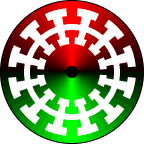Click to Close this View The stator windings of an ac induction motor are distributed around the stator to produce a roughly sinusoidal distribution. When three phase ac voltages are applied to the stator windings, a rotating magnetic field is produced. The rotor of an induction motor also consists of windings or more often a copper squirrel cage embedded within iron laminates. Only the iron laminates are shown. An electric current is induced in the rotor bars which also produce a magnetic field. The rotating magnetic field of the stator drags the rotor around. The rotor does not quite keep up with the rotating magnetic field of the stator. It falls behind or slips as the field rotates. In this animation, for every time the magnetic field rotates, the rotor only makes ¾ of a turn. If you follow one of the bright green or red rotor teeth with the mouse, you will notice it change color as it falls behind the rotating field. The slip has been greatly exaggerated to enable visualization of this concept. A real induction motor only slips a few percent.

The induction machine is modeled at the component level by a set of non-linear causal mathematical relations, which have parameters, which are dependent on the angle between the stator and the rotor of the induction machine.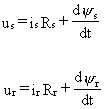where us and ur are the stator and rotor voltages on the machine terminals. The three phase currents are represented by is for the stator currents and ir the rotor currents.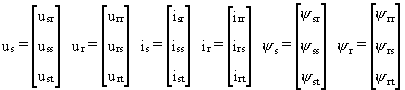The matrices Rs and Rr represent the winding resistance in the stator and rotor for each phase. The fluxes of the machine are presented by: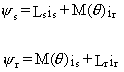with Ls and M(θ) being the self and mutual inductance of the stator and rotor. The matrices Ls and M(θ) are given by: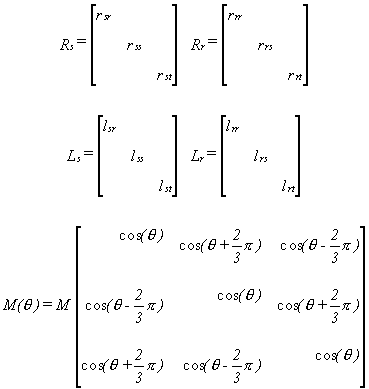In Caspoc the mutual inductance and the leakage inductance are parameters for the IM models. The total stator inductance for the stator equals the Caspoc parameters Lm+Ls, being the sum of the mutual Lm and leakage inductance Ls. The total rotor inductance for the rotor equals the Caspoc parameters Lm+Lr, being the sum of the mutual Lm and leakage inductance Lr. The mutual inductance Lm is the same parameter for both the stator and rotor. In the above matrices, a constant value M and the rotor angle dependent matrix model the mutual inductance.

The dependency of the rotor resistance Rr on the slip of induction machine is modeled by the coupling between the stator and rotor windings. Therefore the value entered in Caspoc for Rr equals the rotor resistance during standstill. The stator resistance Rs is the DC winding resistance of the stator windings.

The number of pole pairs is given by the parameter p. Default p is set to 1. The machine will rotate with nearly the same frequency as the supplying grid for p=1.

The torque produced by the machine is given by: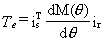The stator and rotor currents is and ir are considered to be state variables with three entries. The input for the equations are the stator and rotor voltages us and ur and the angular position θ of the rotor axis. The output is given by the electric torque Te. Using MISO (Multi Input Single Output) blocks, the equations for the induction machine are modeled in a block-diagram model.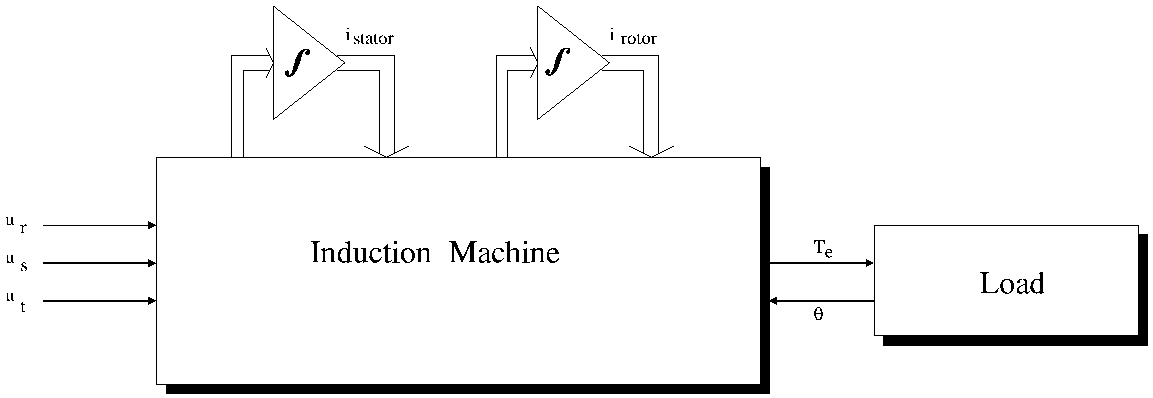Figure: Induction machine block diagram model.

The load of the induction machine is modeled at the component level. Only the friction and the inertia of the load are modeled given by: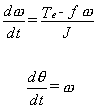This equation is modeled in a block-diagram model shown below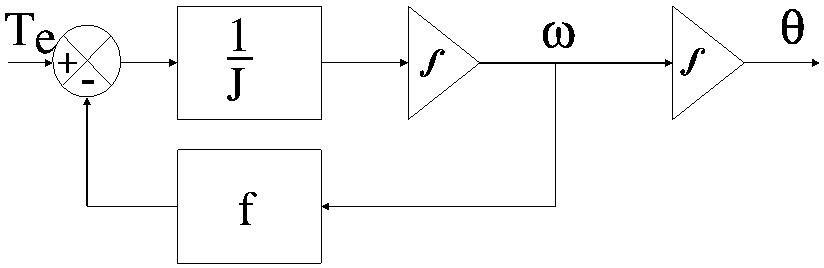Figure: Block-diagram model of the mechanical load.

The block-diagram model of the mechanical load is coupled to the model of the induction machine by means of Te and θ.

In Caspoc the blocks ASMSC and EM are used as the basis for modeling induction machines. The ASMSC is used for modeling squirrel cage motors. Therefor only the stator can be connected to the grid. For other types of the induction machine, the block EM will be used. The library blocks for the induction machines have electrical and mechanical connections. The electrical terminals are dependent on the type of the induction machine.

The mechanical shaft is modeled by a rigid shaft model. Most machine models have internal parameters for modeling the inertia and friction of the shaft. The induction machine can either run without load or can be connected to a mechanical rotational model. Use the models from components/circuit/rotational and from the various mechanical libraries for modeling the mechanical model.